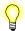# log10(), log10f(), log10l()

Compute the logarithm (base 10) of a number

## Synopsis:

```#include <math.h>

double log10( double x );

float log10f( float x );

long double log10l( long double x );```

## Arguments:

x
The number that you want to compute the log of.

## Library:

libm

Use the -l m option to qcc to link against this library.

## Description:

These functions compute the base 10 logarithm of x:

log10 x

A domain error occurs if x is negative. A range error occurs if x is zero.

## Returns:

The base 10 logarithm of x. For finite values of x less than zero, these functions return NaN. The return value when x is -Inf is NaN.If an error occurs, these functions return 0, but this is also a valid mathematical result. If you want to check for errors, set errno to 0, call the function, and then check errno again. These functions don't change errno if no errors occurred.

## Examples:

```#include <stdio.h>
#include <stdlib.h>
#include <math.h>

int main( void )
{
printf( "%f\n", log10(.5) );

return EXIT_SUCCESS;
}```

produces the output:

`-0.301030`

## Classification:

Safety:
Cancellation point No
Interrupt handler No
Signal handler No